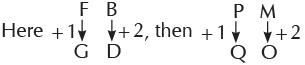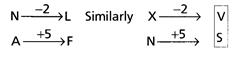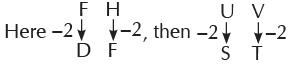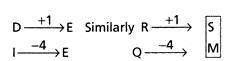Courses

# Olympiad Test: Series And Pattern - 2

## 20 Questions MCQ Test Mathematics Olympiad Class 4 | Olympiad Test: Series And Pattern - 2

Description
This mock test of Olympiad Test: Series And Pattern - 2 for Class 4 helps you for every Class 4 entrance exam. This contains 20 Multiple Choice Questions for Class 4 Olympiad Test: Series And Pattern - 2 (mcq) to study with solutions a complete question bank. The solved questions answers in this Olympiad Test: Series And Pattern - 2 quiz give you a good mix of easy questions and tough questions. Class 4 students definitely take this Olympiad Test: Series And Pattern - 2 exercise for a better result in the exam. You can find other Olympiad Test: Series And Pattern - 2 extra questions, long questions & short questions for Class 4 on EduRev as well by searching above.
QUESTION: 1

### Identify the one that does not belong to the group.

Solution:

Roof is different as other three are parts of a tree.

QUESTION: 2

### Identify the one which is different from the others.

Solution:

Math is a subject.

QUESTION: 3

### Find the odd one out.

Solution:
QUESTION: 4

Which one is different from others?

Solution:
QUESTION: 5

Identify the one that does not belong to the group.

Solution:
QUESTION: 6

Direction: Find the letter which will end the first word and start the second word.
BE?AS

Solution:

BEG and GAS

QUESTION: 7

Direction: Find the letter which will end the first word and start the second word.
BOT?URT

Solution:
QUESTION: 8

Direction: Find the letter which will end the first word and start the second word.
SHAR?RINT

Solution:
QUESTION: 9

Direction: Find the letter which will end the first word and start the second word.
CAME?IGHT

Solution:
QUESTION: 10

Direction: Find the letter which will end the first word and start the second word.
HAN?ATE

Solution:
QUESTION: 11

Direction: Find the letter which will end the first word and start the second word.
SAL?ILL

Solution:
QUESTION: 12

Direction: Find the letter which will end the first word and start the second word.
MA?ET

Solution:
QUESTION: 13

Direction: Find the letter which will end the first word and start the second word.
DUC?ITE

Solution:
QUESTION: 14

Direction: Find the letter which will end the first word and start the second word.
MAT?VER

Solution:
QUESTION: 15

Direction: Find the letter which will end the first word and start the second word.
RUI?EAR

Solution:
QUESTION: 16

Direction: In each of the following questions which alternative will replace the question mark.
FB is to GD as PM is to ?

Solution:QUESTION: 17

Direction: In each of the following questions which alternative will replace the question mark.
NA is to LF as XN is to?

Solution:QUESTION: 18

Direction: In each of the following questions which alternative will replace the question mark.
FH is to DF as UV is to?

Solution:QUESTION: 19

Direction: In each of the following questions which alternative will replace the question mark.
KM is to OJ as VH is to ?

Solution:
QUESTION: 20

Direction: In each of the following questions which alternative will replace the question mark.
DI is to EE as RQ is to?

Solution: